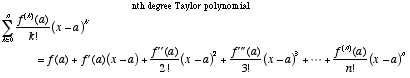index: click on a letter A B C D E F G H I J K L M N O P Q R S T U V W X Y Z A to Z index index: subject areas numbers & symbols sets, logic, proofs geometry algebra trigonometry advanced algebra & pre-calculus calculus advanced topics probability & statistics real world applications multimedia entrieswww.mathwords.com about mathwords website feedback

 Taylor Polynomial nth Degree Taylor Polynomial An approximation of a function using terms from the function's Taylor series. An nth degree Taylor polynomial uses all the Taylor series terms up to and including the term using the nth derivative.See also